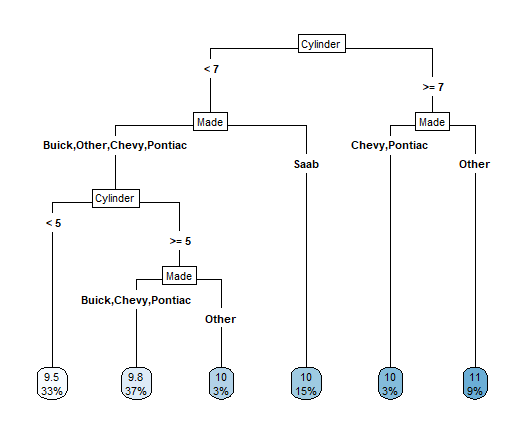# Using flashlight with mlr3

#### 2021-04-20

library(dplyr)
library(MetricsWeighted)
library(flashlight)
library(caret)
library(mlr3)
library(mlr3learners)

## Introduction

This vignette shows how to use flashlight for interpretation of models trained with mlr3. It is very straightforward - no extra line of code is required.

## Training a linear regression and a random forest on the cars data set from package caret

data(cars)
str(cars)
#> 'data.frame':    804 obs. of  18 variables:
#>  $Price : num 22661 21725 29143 30732 33359 ... #>$ Mileage    : int  20105 13457 31655 22479 17590 23635 17381 27558 25049 17319 ...
#>  $Cylinder : int 6 6 4 4 4 4 4 4 4 4 ... #>$ Doors      : int  4 2 2 2 2 2 2 2 2 4 ...
#>  $Cruise : int 1 1 1 1 1 1 1 1 1 1 ... #>$ Sound      : int  0 1 1 0 1 0 1 0 0 0 ...
#>  $Leather : int 0 0 1 0 1 0 1 1 0 1 ... #>$ Buick      : int  1 0 0 0 0 0 0 0 0 0 ...
#>  $Cadillac : int 0 0 0 0 0 0 0 0 0 0 ... #>$ Chevy      : int  0 1 0 0 0 0 0 0 0 0 ...
#>  $Pontiac : int 0 0 0 0 0 0 0 0 0 0 ... #>$ Saab       : int  0 0 1 1 1 1 1 1 1 1 ...
#>  $Saturn : int 0 0 0 0 0 0 0 0 0 0 ... #>$ convertible: int  0 0 1 1 1 1 1 1 1 0 ...
#>  $coupe : int 0 1 0 0 0 0 0 0 0 0 ... #>$ hatchback  : int  0 0 0 0 0 0 0 0 0 0 ...
#>  $sedan : int 1 0 0 0 0 0 0 0 0 1 ... #>$ wagon      : int  0 0 0 0 0 0 0 0 0 0 ...

We then use the data to fit two types of regression to predict log(Price) by log(Mileage) and the other covariables. Car mades are already dummy coded. We will revert this in order to simplify the explainer process. Additionally, we represent some 0-1 dummies by nice, meaningful factors.

undo_dummies <- function(df, cols) {
factor(data.matrix(df[, cols]) %*% seq_along(cols), labels = cols)
}

no_yes <- function(x) {
factor(x, 0:1, c("no", "yes"))
}

# Prepare data
cars <- cars %>%
mutate(Price = log(Price),
Mileage = log(Mileage),
mutate_at(c("Cruise", "Sound", "Leather"), no_yes)

# Response and covariables
y <- "Price"
x <- c("Cylinder", "Doors", "Cruise", "Sound", "Leather", "Mileage", "Made")

# Data split
set.seed(1)
idx <- c(createDataPartition(cars[[y]], p = 0.7, list = FALSE))
tr <- cars[idx, c(y, x)]
te <- cars[-idx, c(y, x)]

task_cars <- TaskRegr$new(id = "cars", backend = tr, target = y) # Fit the models fit_lm <- lrn("regr.lm") fit_lm$train(task_cars)
fit_rf$train(task_cars) ## flashlights Then we collect all infos to build (multi-)flashlights, the core objects for explaining and comparing the models. fl_lm <- flashlight(model = fit_lm, label = "lm") fl_rf <- flashlight(model = fit_rf, label = "rf") fls <- multiflashlight(list(fl_lm, fl_rf), y = y, data = te, metrics = list(RMSE = rmse, R-Squared = r_squared)) ## Explaining the models Let us go through a selection of explainability tools. ### Performance The models perform essentially similar. light_performance(fls) %>% plot(fill = "darkred")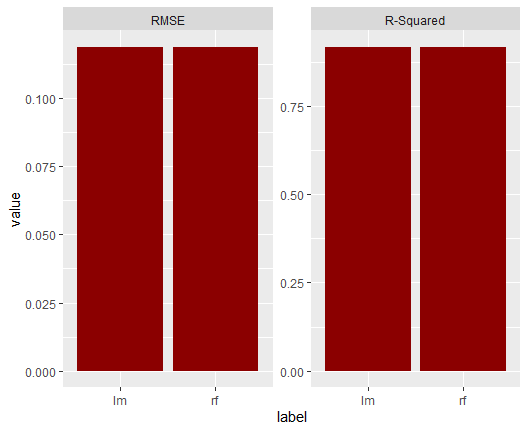### Importance Let’s study permutation importance regarding RMSE metric. imp <- light_importance(fls) plot(imp, fill = "darkred")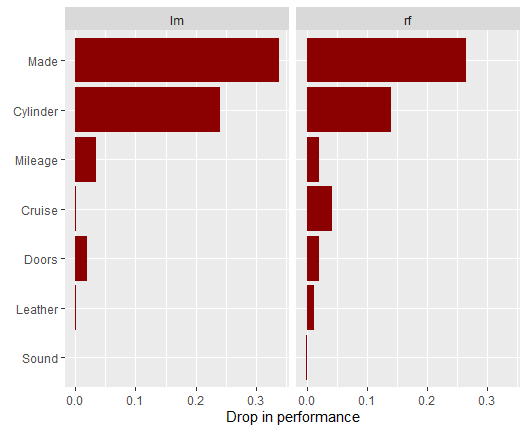### Effects Now, let’s look at a couple of ways to visualize effects. # Individual conditional expectations (ICE). Using a seed guarantees the same observations across models light_ice(fls, v = "Cylinder", n_max = 100, seed = 54) %>% plot(alpha = 0.1)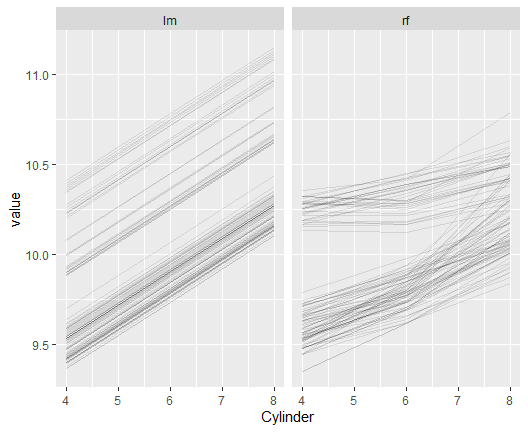# Partial dependence profiles light_profile(fls, v = "Cylinder") %>% plot()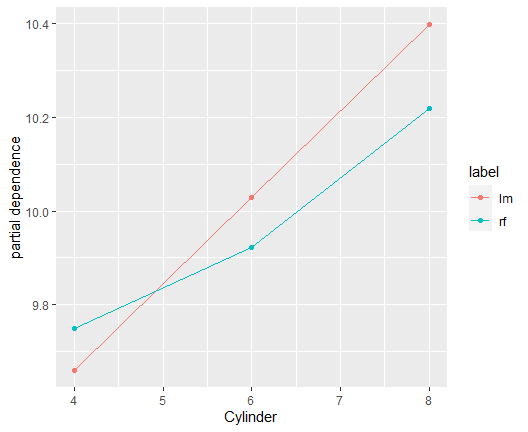light_profile(fls, v = "Cylinder", by = "Leather") %>% plot()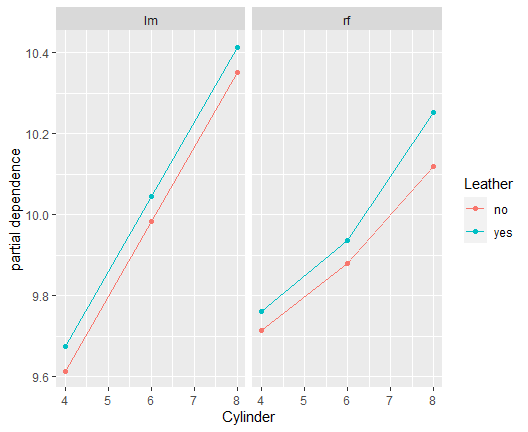# Accumulated local effects light_profile(fls, v = "Cylinder", type = "ale") %>% plot()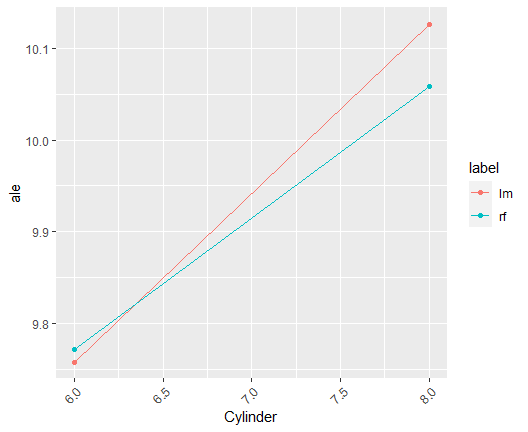# M-Plots light_profile(fls, v = "Mileage", type = "predicted") %>% plot()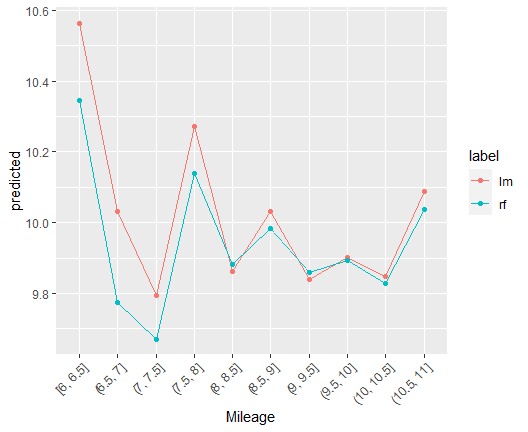# Response profiles, prediction profiles, partial dependence in one eff <- light_effects(fls, v = "Cylinder") eff %>% plot() %>% plot_counts(eff, alpha = 0.3)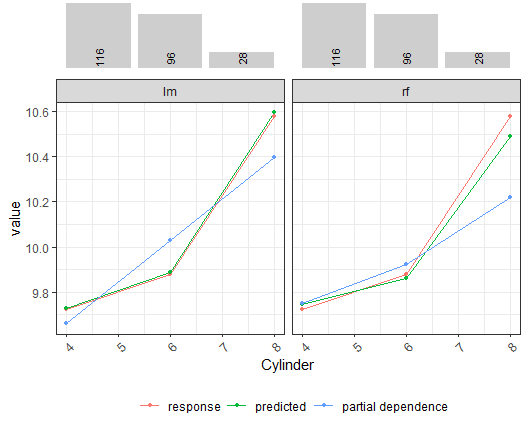### Interaction strength How strong are the pairwise interactions among the four most important predictors? Surprise, surprise: for the linear regression, there are none! light_interaction(fls, v = most_important(imp, 4), pairwise = TRUE, n_max = 50, seed = 63) %>% plot(fill = "darkred")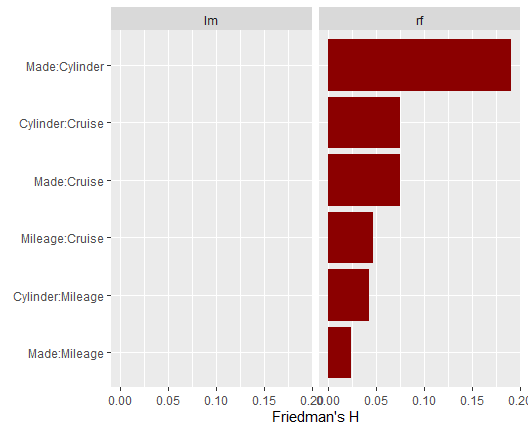### Variable contribution breakdown for single observation We can explain the predictions of single observations… light_breakdown(fls, new_obs = te[1, ]) %>% plot(size = 3, facet_ncol = 2)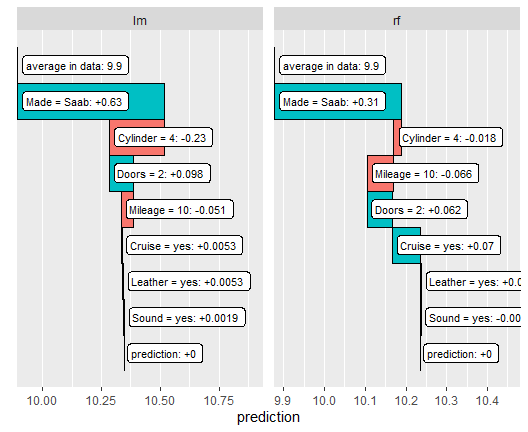### Global surrogate Let’s approximate the random forest model by a simple decision tree. light_global_surrogate(fls$rf) %>%
plot()